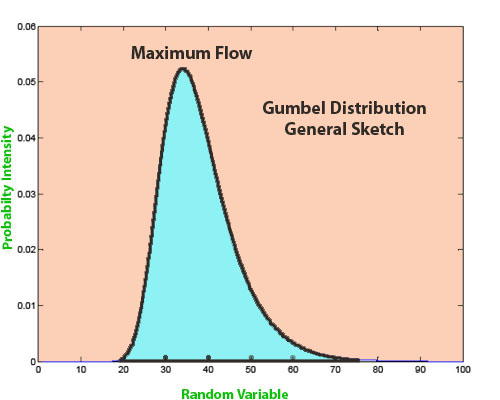Feb 26, 2016

Feb 26, 2016

Feb 26, 2016

# Flood Frequency Analysis - Gumbel MethodFlood Frequency Analysis is the statistical calculation of the probability of occurrence of flood of specific magnitude in a river, the specific period is called return period and the flow can be ranked as maximum possible flow. In Hydrology, flow frequency analysis has occupied an important role and can be effectively used in any hydrology related project. A Flow frequency study is required to be carried out to evaluate the high flows for different return periods by using frequency analysis techniques.

## Methods for Flow Frequency Analysis

Different types of methods are used for flow frequency analysis.

• Gumble method,
• Pearson type-III method
• Log person method
• Normal method
• Log Normal distributions.

For analysis, maximum flow can be established from the flow record available near by the gauging station at project site. For every high flow period one maximum value is obtained for analysis.The series of maximum flows can be analyzed using various frequency distribution methods (Gumble, Type III External Distribution, Pearson Type III, log Normal). The Results obtained from the analysis of the above method can be adopted for flow analysis. Gumble distribution is a method used to form the distribution of the maximum or minimum of a number of flows obtained. The observed maximum/minimum flows can be categorized from the highest to the lowest value and a plotting position was assigned according to the weibull formula.

P = m/(n + 1)

Where, P = probability and plotting position m= is the order of the minimum flow in the array. n = number of years of the record.